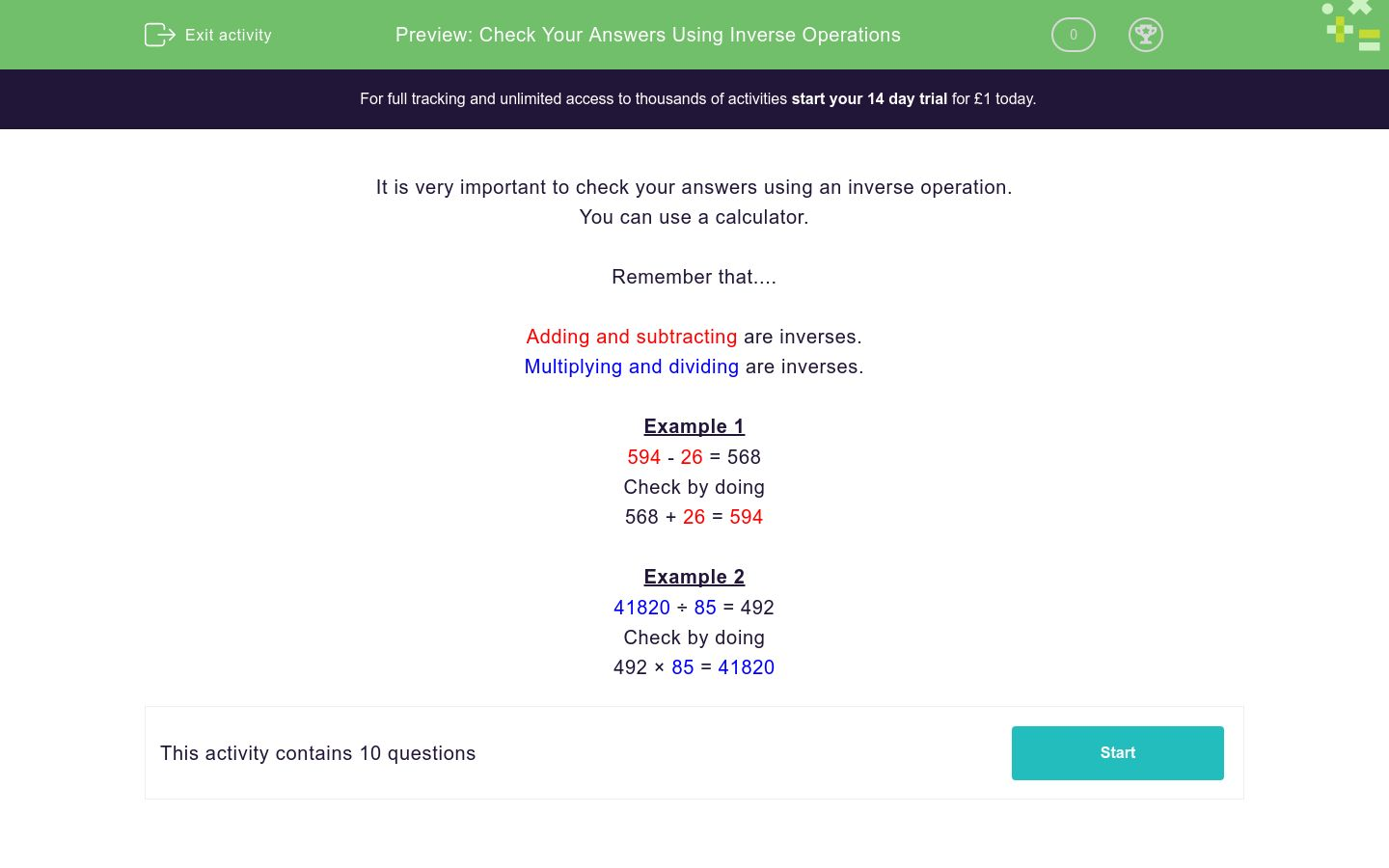### Affordable pricing from £10/month

In this worksheet, students check their answers with a calculator by using the correct inverse operation.Key stage:  KS 3

Curriculum topic:   Number

Curriculum subtopic:   Use Relationships Between Operations

Difficulty level:### QUESTION 1 of 10

It is very important to check your answers using an inverse operation.

You can use a calculator.

Remember that....

Multiplying and dividing are inverses.

Example 1

594 - 26 = 568

Check by doing

568 + 26 = 594

Example 2

41820 ÷ 85 = 492

Check by doing

492 × 85 = 41820

Check your results of the following by doing an inverse operation.

5907 × 0.87 =

Check your results of the following by doing an inverse operation.

2962 × 0.23 =

Check your results of the following by doing an inverse operation.

3326 × 0.3 =

Check your results of the following by doing an inverse operation.

9140 - 59 =

Check your results of the following by doing an inverse operation.

4440 ÷ 0.2 =

Check your results of the following by doing an inverse operation.

758 - 68 =

Check your results of the following by doing an inverse operation.

3359 × 0.9 =

Check your results of the following by doing an inverse operation.

5396 × 0.24 =

Check your results of the following by doing an inverse operation.

1.7 + 6.48 =

Check your results of the following by doing an inverse operation.

7 + 8.44 =
• Question 1

Check your results of the following by doing an inverse operation.

5907 × 0.87 =
5139.09
• Question 2

Check your results of the following by doing an inverse operation.

2962 × 0.23 =
681.26
• Question 3

Check your results of the following by doing an inverse operation.

3326 × 0.3 =
997.8
• Question 4

Check your results of the following by doing an inverse operation.

9140 - 59 =
9081
• Question 5

Check your results of the following by doing an inverse operation.

4440 ÷ 0.2 =
22200
• Question 6

Check your results of the following by doing an inverse operation.

758 - 68 =
690
• Question 7

Check your results of the following by doing an inverse operation.

3359 × 0.9 =
3023.1
• Question 8

Check your results of the following by doing an inverse operation.

5396 × 0.24 =
1295.04
• Question 9

Check your results of the following by doing an inverse operation.

1.7 + 6.48 =
8.18
• Question 10

Check your results of the following by doing an inverse operation.

7 + 8.44 =
15.44
---- OR ----

Sign up for a £1 trial so you can track and measure your child's progress on this activity.

### What is EdPlace?

We're your National Curriculum aligned online education content provider helping each child succeed in English, maths and science from year 1 to GCSE. With an EdPlace account you’ll be able to track and measure progress, helping each child achieve their best. We build confidence and attainment by personalising each child’s learning at a level that suits them.

Get started